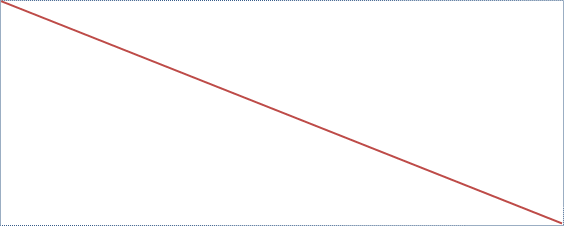PowerPoint tips, hints and tutorials that will change your presentations for ever!

INDEXPowerPoint vba - End Co-ordinates of a Line

When you draw a line with vba you will specify the start and end co-rdinates x1,y1,x2 and y2.

However if you are given a predrawn line it is not obvious how to find those co-ordinates!

The first hint is to treat the line as having a bounding box.It is then not too difficult to use the left, top, width and height to deduce the co-ordinates.

EXCEPT:

How do you know the line is not like this:Clearly in this direction the co-ordinates of the ends will be completely different.

It's not obvious that the line has any property which will tell you the slope.

Here's How

In the first example the bounding box has been drawn top left to bottom right. The "Normal" way! The box has two properties FlipHorizontal and FlipVertical. In the first "Normal" example both will be set to False.

The second example has been drawn from the bottom right and is considered to be vertically flipped. In this example FlipHorizontal will still be False but FilpVertical will be True.

If we draw the line (and therefore the bounding box) right to left then it is considered orizontally flipped.

There are therefore four combinations of VerticalFilp and Horizontal Flip which tell us in which direction the line was drawn and therefore how to deduce the co-ordinates.

This Function will tell you which direction the line was drawn and help you find the co-ordinates.

``````Function Line_Direction(oLine As Shape) As String
If oLine.AutoShapeType=-2 or oLine.Type=msoLine Then
If oLine.Width = 0 Then
If oLine.VerticalFlip = True Then
Line_Direction = "VerticalUP"
Else
Line_Direction = "VerticalDOWN"
End If
Exit Function
End If
If oLine.Height = 0 Then
If oLine.HorizontalFlip = False Then
Line_Direction = "HorizontalRIGHT"
Else
Line_Direction = "HorizontalLEFT"
End If
Exit Function
End If

Select Case oLine.VerticalFlip
Case Is = True
If oLine.HorizontalFlip = False Then
Line_Direction = "Diagonal RIGHT UP"
Else: Line_Direction = "Diagonal LEFT UP"
End If
Case Is = False
If oLine.HorizontalFlip = False Then
Line_Direction = "Diagonal RIGHT DOWN"
Else: Line_Direction = "Diagonal LEFT DOWN"
End If
End Select
Else
Line_Direction = "NOT A LINE"
End If
End Function``````

To Use the Function to Get the Direction of a Selected Line Use:

Sub getDirection()
MsgBox Line_Direction(ActiveWindow.Selection.ShapeRange(1))
End Sub

Back to the Index Page